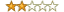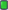Thread: Find the Algorithm View Single Post
#6
 chantsFamilyJoin Date: Jul 2016 Posts: 530 Rept. Given: 5 Rept. Rcvd 35 Times in 21 Posts Thanks Given: 441 Thanks Rcvd at 793 Times in 370 PostsJust open a Python 3.7 console:
Code:
```>>> x=0x0101
>>> hex((x&0xf==1)*0x70f5+(x&0xf==0)*0x2c8c+(x&0xf==2)*0xdde2+(x&0xf==0xf)*0x5967)
'0x70f5'
>>> x=0xde50
>>> hex((x&0xf==1)*0x70f5+(x&0xf==0)*0x2c8c+(x&0xf==2)*0xdde2+(x&0xf==0xf)*0x5967)
'0x2c8c'
>>> x=0x0102
>>> hex((x&0xf==1)*0x70f5+(x&0xf==0)*0x2c8c+(x&0xf==2)*0xdde2+(x&0xf==0xf)*0x5967)
'0xdde2'
>>> x=0xffff
>>> hex((x&0xf==1)*0x70f5+(x&0xf==0)*0x2c8c+(x&0xf==2)*0xdde2+(x&0xf==0xf)*0x5967)
'0x5967'```
The formula is
Code:
`f(x)=(x&0xf==1)*0x70f5+(x&0xf==0)*0x2c8c+(x&0xf==2)*0xdde2+(x&0xf==0xf)*0x5967`
You should learn how and why this works and try to understand the basic code. Its not the solution you are looking for but simply asking for a mapping function with a few points is pretty generic. Without having some white box information or a lot of values or some obvious pattern, my answer could be as good as any.
 The Following 2 Users Say Thank You to chants For This Useful Post: Abaddon (01-19-2020), mcr4ck (01-18-2020)